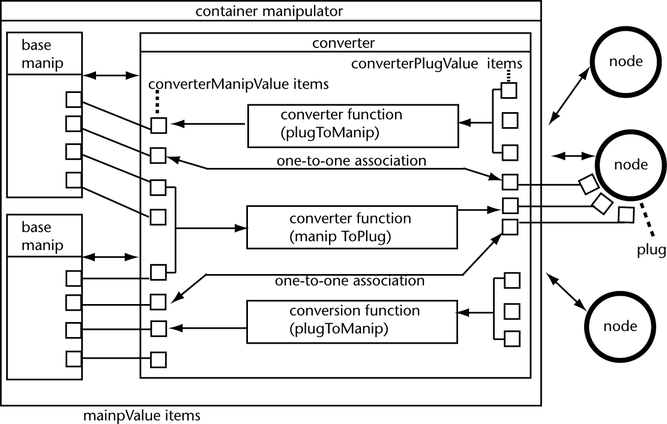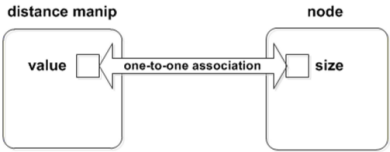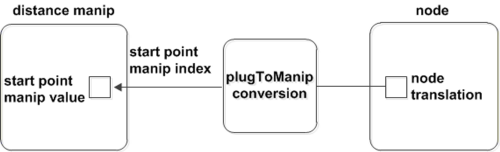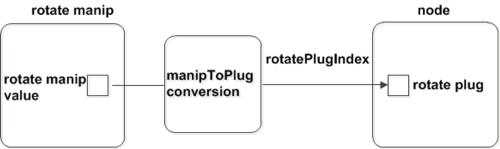﻿ Communication Between Manipulators and Nodes

# Communication Between Manipulators and Nodes

Manipulators communicate with plugs on nodes to set the values of the plugs. Additionally, manipulators also set the manipulator values with respect to the values of the plugs.

The communication between manipulators and nodes is done in one of these two ways: simple one-to-one associations, or through complex conversion functions. The following diagram illustrates the communication between nodes and manipulators.The converter manages the communication between the plugs on the nodes and manipulator values. The arrows in the diagram indicate the direction in which the information flows. Each container manipulator has one converter, which is the interface between the children manipulators and the affected plugs.

The square boxes indicate the data items on the converter and the base manipulators. The items on the converter that are related to the children manipulator values are called the converterManipValue items. The items on the converter that are related to the node plug values are called the converterPlugValue items.

The items on the base manipulators are called the manipValue items. Some manipValue items relate directly to an affordance of the manipulator. For example, MFnDiscManip::angleIndex() relates directly to the rotation affordance of the DiscManip. Other manipValue items do not relate to an affordance of the manipulator, but provide important information on the position or orientation of the manipulator such as MFnDiscManip::centerIndex() and MFnDiscManip::axisIndex().

Each converterManipValue item and converterPlugValue item has an integer index to uniquely identify the item. Each manipValue item on a base manipulator also has an integer index to uniquely identify the item.

NOTE: There is a one-to-one correspondence between a converterManipValue item and a base manipulator’s manipValue item, and these items share the same integer index. There is also a one-to-one correspondence between a converterPlugValue item and a plug on a node, which is affected by the manipulator.

As shown in the diagram, there are one-to-one associations directly between a converterManipValue item and a converterPlugValue item.

More complex conversions between converterManipValue items and converterPlugValue items are performed through conversion functions. These functions can use any number of converterPlugValue items or converterManipValue items to calculate the corresponding converterManipValue or converterPlugValue item.

## One-to-one Association

One-to-one association synchronizes the manipulator values with the plug values. In other words, the manipulator value equals the plug value, and vice versa.

One-to-one associations between a converterManipValue item and a converterPlugValue item are established through methods on the manipulator classes derived from MFnManip3D.These methods and the data types corresponding to the plugs they connect to are the following:

These methods must be called from the connectToDependNode() method. For example, in the footPrintManip example:

```MStatus footPrintLocatorManip::connectToDependNode(const MObject &node)

{
...
MFnDistanceManip distanceManipFn(fDistanceManip);
MFnDependencyNode nodeFn(node);
MPlug sizePlug = nodeFn.findPlug("size", &stat);
if (MStatus::kFailure != stat) {
distanceManipFn.connectToDistancePlug(sizePlug);
...
MPxManipContainer::connectToDependNode(node);
}
return stat;
}
```

The following figure shows the one-to-one association between the distance manipulator value and the value of the size plug on a node.After you set the one-to-one association, whenever the manipulator value changes, the corresponding plug value also changes, and vice versa.

One-to-one association is a convenient approach to set the relationships between manipulator values and plug values. However, it might not be useful for more complex requirements. For example, you cannot set the distance manipulator value to 5 x the value of size. Also, when you move the node, the distance manipulator will not appear at the center of the node, and you cannot move the manipulator along the position of the node. If the position of a manipulator needs to be affected by the position of an object, or a group of manipulators has to move together in a specific way, then you need to use conversion functions.

## Conversion Functions

Conversion functions are used to convert between manipulator values and plug values. They are implemented as callback methods. Conversion functions are used to set complex relationships between manipulator values and plug values.

A simple example of a manipulator that uses conversion functions is a container manipulator with a DiscManip connected to a plug (associated with an attribute of type MFnUnitAttribute::kAngle) that takes the rotation of the disc manipulator and multiplies that rotation by 10. The conversion function uses MPxManipContainer::getConverterManipValue() on MFnDiscManip::angleIndex() and then multiplies that angle by 10.

Conversion functions are very useful when the position of a manipulator has to be affected by the position of an object, or to move a group of manipulators together in a specific way. Without conversion functions, manipulators cannot move together as a unit, and certain components of the manipulator will remain either at the origin or a fixed position in space.

NOTE: Conversion functions are not required when a FreePointTriadManip specifies a position or a PointOnCurveManip specifies a parameter along a curve. However, if you have a DiscManip that you want to move along with the PointOnCurveManip, you need a conversion function to give the DiscManip information about its position and normal.

There are two kinds of conversion callback methods: plugToManip and manipToPlug.

### Conversion Callback - plugToManip

A plugToManip conversion callback is used to get the value of a converterManipValue item from various converterPlugValue items. This callback has access to all the converterPlugValue items and returns the value of a converterManipValue item.

The plugToManip conversion is implemented by calling MPxManipContainer::addPlugToManipConversionCallback().

Each visual control property has a corresponding index registered with the manipulator. To set the plugToManip conversion relationship, when MPxManipContainer::addPlugToManipConversionCallback() is called, the index of the manipulator value must be passed. In each base manipulator function set class, there are functions to retrieve different indices for individual properties.

The following code from footPrintManip illustrates how to retrieve the index of the starting point of the manipulator and pass it to the addPlugToManipConversionCallback() function call. The second parameter of this function call is the callback conversion function, which calculates the manipulator value of the starting point based on some plug values.

```unsigned startPointIndex = distanceManipFn.startPointIndex();

The plugToManip conversion callback accesses the plug values, calculates the manipulator values based on the custom algorithm, and returns them. In this example, the callback function does not request the plug values, instead, it retrieves the node translation in world space and returns this value. By doing so, it actually sets the start point manipulator value to be the same as the node translation. The result is that whenever the node moves, the manipulator moves along with it, and always appears in the center of the node.

```MManipData footPrintLocatorManip::startPointCallback(unsigned index) const {
MFnNumericData numData;
MObject numDataObj =numData.create(MFnNumericData::k3Double);
MVector vec = nodeTranslation();
numData.setData (vec.x, vec.y, vec.z);
return(MManipData (numDataObj));
}```The conversion callback returns a data type called MManipData and sets the manipulator value to what the MManipData represents.

### Conversion Callback - manipToPlug

A manipToPlug conversion callback is used to get the value of a converterPlugValue item from various converterManipValue items. This callback has access to all the converterManipValue items and returns the value of a converterPlugValue item.

The manipToPlug conversion is implemented by calling MPxManipContainer::addManipToPlugConversionCallback(). The corresponding plug needs to be passed in as the first parameter because the callback needs to know which plug values to change.

The following code from the rotateManip example demonstrates using this technique with the node's rotate plug passed in.

```MFnDependencyNode nodeFn (node);
MPlug rPlug =nodeFn.findPlug ("rotate", &stat);
…

The addManipToPlugConversionCallback() function call returns the index rotatePlugIndex to identify which plug is registered with this callback. When the actual callback function is invoked, the index of the plug value to be calculated is passed in as the parameter of the function call. The callback function then determines if the passed-in value is the index that is registered for this callback. If there is more than one plug registered with this callback, a condition statement can be used to distinguish different passed-in plug index, and calculate corresponding manipulator values. In the following rotationChangedCallback() example, the passed-in index is compared with rotatePlugIndex. If they are equal, getConverterManipValue() is called with rotate manipulator index to retrieve the rotate manipulator value. By returning the rotate manipulator value as a MManipData object, this callback function is setting the rotate plug value to be the same as the rotate manipulator value. Depending on your specific requirement, more complex relationship between rotate plug value and rotate manipulator value can be set using conversion callback.

```MManipData exampleRotateManip::rotationChangedCallback(unsigned index) {
MObject obj =MObject::kNullObj;
// If we entered the callback with an invalid index, print an error and
// return.  Because, we registered the callback only for one plug, all
// invocations of the callback must be for that plug.
if (index != rotatePlugIndex) {
MGlobal::displayError("Invalid index in rotation changed callback!");
// For invalid indices, return vector of 0's
MFnNumericData numericData;
obj = numericData.create(MFnNumericData::k3Double);
numericData.setData (0.0, 0.0, 0.0);
return (obj);
}
MFnNumericData numericData;
obj =numericData.create(MFnNumericData::k3Double);
MEulerRotation manipRotation;
if (!getConverterManipValue(rotateManip.rotationIndex(),manipRotation)) {
MGlobal::displayError("Error retrieving manip value");
numericData.setData(0.0, 0.0, 0.0);
} else {
numericData.setData(manipRotation.x, manipRotation.y, manipRotation.z);
}
return MManipData(obj);
}```In general, manipToPlug conversions are not used commonly. In addition to using converterPlugValues and converterManipValues, it is sometimes useful to use class data, such as a DAG path. (See the footPrintManip for an example of how fNodePath is used to calculate the node translation.) For manipulators that operate on components, it might also be useful to store initial component positions (see the componentScaleManip for an example of how this is done).

### MManipData

The conversion callback methods return a data type called MManipData. MManipData encapsulates manipulator data which is returned from the manipulator conversion functions. It represents data that is either simple or complex. The simple data methods on MManipData are used to represent bool, short, long, unsigned, float, and double types.

NOTE: Sometimes attributes associated with the simple data types have higher level meanings such as distance, angle, and time (for example, MFnUnitAttribute::kAngle, MFnUnitAttribute::kDistance, and MFnUnitAttribute::kTime).

MManipData is also used to represent complex data types created by MFnData or classes derived from MFnData, such as matrices, curves, and arrays of data.

The footPrintManip plug-in has an example of a plugToManip conversion callback called startPointCallback. The startPointCallback returns an MManipData which is set to an MObject that is created by MFnNumericData.

```class footPrintLocatorManip : public MPxManipContainer
{
public:
...
MManipData startPointCallback(unsigned index) const;
MVector nodeTranslation() const;
MDagPath fDistanceManip;
...
};
MManipData footPrintLocatorManip::startPointCallback
(unsigned index) const
{
// The index is the startPointIndex that is
// but it is not necessary to use this in the callback.
MFnNumericData numData;
MObject numDataObj =
numData.create(MFnNumericData::k3Double);
MVector vec = nodeTranslation();
numData.setData(vec.x, vec.y, vec.z);
return MManipData(numDataObj);
}
MStatus footPrintLocatorManip::connectToDependNode
(const MObject &node)
{
...
unsigned startPointIndex =
distanceManipFn.startPointIndex();
startPointIndex,
(plugToManipConversionCallback) startPointCallback);
...
}
```

### Tips

Conversion Functions for Python and C++ – For python plug-ins, the only functions that you can use to register conversion callback are: addManipToPlugConversion() and addPlugToManipConversion(). For C++ plug-ins, you can either use the addPlugToManipConversionCallback()/addManipToPlugConversionCallback() functions or addManipToPlugConversion()/addPlugToManipConversion() functions to register conversion callback.

Manip Index and Plug Index – The visual control properties have a manip index registered within the manipulator. For example, MFnDistanceManip::startPointIndex() returns the index of the start point and MFnRotateManip::rotationIndex() returns the index of the rotation manipulator value. To specify which manipulator value is set with a plugToManip conversion callback, the manip index must be used when addPlugToManipConversionCallback() or addPlugToManipConversion() is called.

The plug index is returned by addManipToPlugConversionCallback() and addManipToPlugConversion() to identify which plug is registered with the manipToPlug conversion callback.

In addition, the manip indexes are used to retrieve the manipulator values from the getConverterManipValue() function, and the plug indexes are used to retrieve the plug values from the getConverterPlugValue() function.